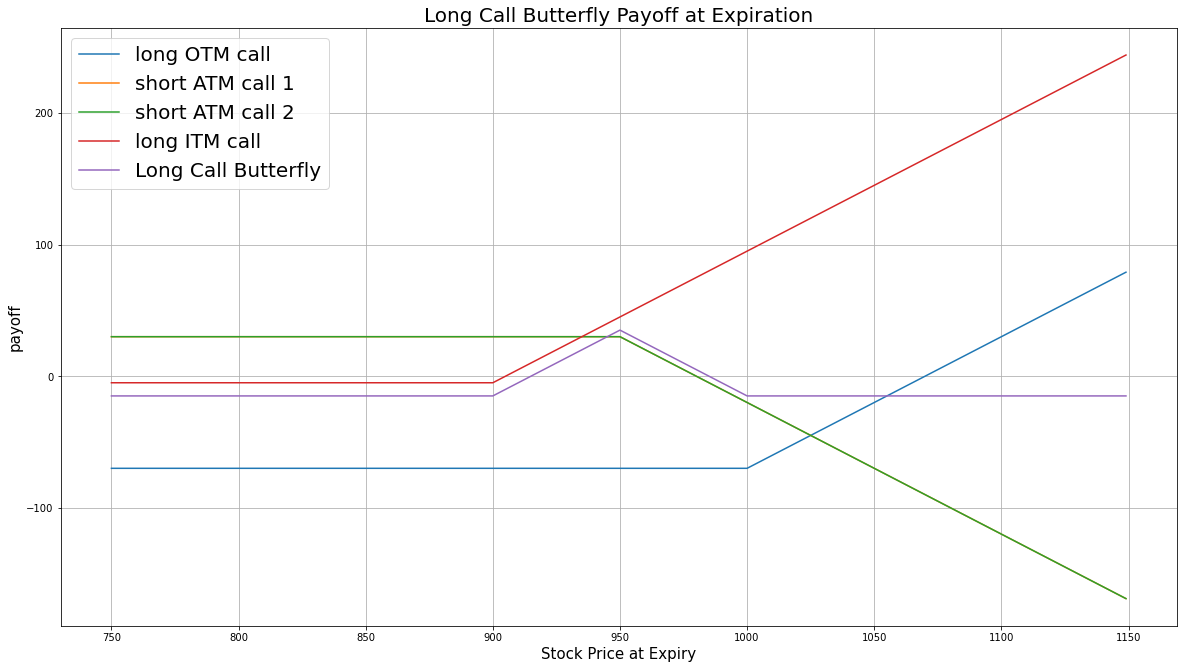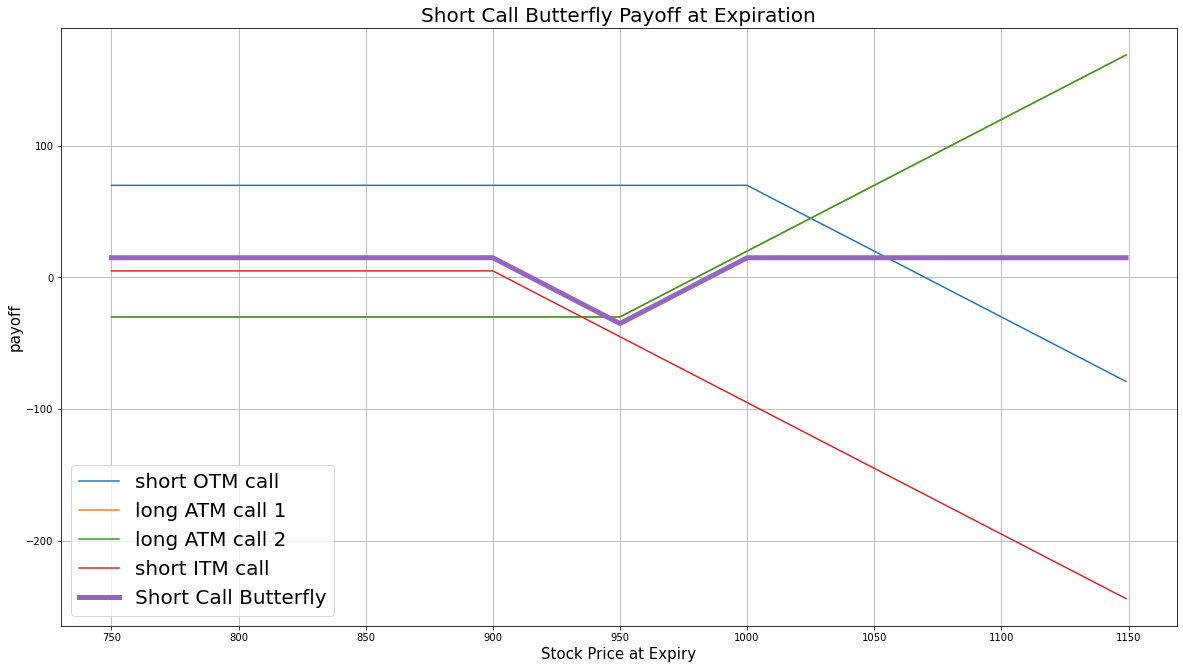# Option Strategies

## Call Butterfly

### Introduction

The call butterfly strategy is the combination of a bull call spread and a bear call spread. In the call butterfly, all of the calls should have the same underlying Equity, the same expiration date, and the same strike price distance between the ITM-ATM and OTM-ATM call pairs. The call butterfly can be long or short.

#### Long Call Butterfly

The long call butterfly consists of a long ITM call, a long OTM call, and 2 short ATM calls. This strategy profits from low volatility in the underlying Equity price.

#### Short Call Butterfly

The short call butterfly consists of a short ITM call, a short OTM call, and 2 long ATM calls. This strategy profits from high volatility in the underlying Equity price.

### Implementation

Follow these steps to implement the call butterfly strategy:

1. In the Initialize method, set the start date, end date, cash, and Option universe.
2. private Symbol _symbol;

public override void Initialize()
{
SetStartDate(2017, 2, 1);
SetEndDate(2017, 3, 5);
SetCash(500000);

_symbol = option.Symbol;
option.SetFilter(universe => universe.IncludeWeeklys()
.Strikes(-15, 15)
.Expiration(TimeSpan.FromDays(0), TimeSpan.FromDays(31)));
}
def Initialize(self) -> None:
self.SetStartDate(2017, 2, 1)
self.SetEndDate(2017, 3, 5)
self.SetCash(500000)

self.symbol = option.Symbol
option.SetFilter(self.UniverseFunc)

def UniverseFunc(self, universe: OptionFilterUniverse) -> OptionFilterUniverse:
return universe.IncludeWeeklys().Strikes(-15, 15).Expiration(timedelta(0), timedelta(31))
3. In the OnData method, select the expiration and strikes of the contracts in the strategy legs.
4. public override void OnData(Slice slice)
{
if (Portfolio.Invested) return;

// Get the OptionChain
var chain = slice.OptionChains.get(_symbol, null);
if (chain == null || chain.Count() == 0) return;

// Get the furthest expiry date of the contracts
var expiry = chain.OrderByDescending(x => x.Expiry).First().Expiry;

// Select the call Option contracts with the furthest expiry
var calls = chain.Where(x => x.Expiry == expiry && x.Right == OptionRight.Call);
if (calls.Count() == 0) return;

// Get the strike prices of the all the call Option contracts
var callStrikes = calls.Select(x => x.Strike).OrderBy(x => x);

// Get the ATM strike price
var atmStrike = calls.OrderBy(x => Math.Abs(x.Strike - chain.Underlying.Price)).First().Strike;

// Get the strike prices for the contracts not ATM
var spread = Math.Min(Math.Abs(callStrikes.First() - atmStrike), Math.Abs(callStrikes.Last() - atmStrike));
var itmStrike = atmStrike - spread;
var otmStrike = atmStrike + spread;
def OnData(self, slice: Slice) -> None:
if self.Portfolio.Invested: return

# Get the OptionChain
chain = slice.OptionChains.get(self.symbol, None)
if not chain: return

# Get the furthest expiry date of the contracts
expiry = sorted(chain, key = lambda x: x.Expiry, reverse=True).Expiry

# Select the call Option contracts with the furthest expiry
calls = [i for i in chain if i.Expiry == expiry and i.Right == OptionRight.Call]
if len(calls) == 0: return

# Get the strike prices of the all the call Option contracts
call_strikes = sorted([x.Strike for x in calls])

# Get the ATM strike price
atm_strike = sorted(calls, key=lambda x: abs(x.Strike - chain.Underlying.Price)).Strike

# Get the strike prices for the contracts not ATM
spread = min(abs(call_strikes - atm_strike), abs(call_strikes[-1] - atm_strike))
otm_strike = atm_strike + spread
5. In the OnData method, call the OptionStrategies.CallButterfly method and then submit the order.
6. var optionStrategy = OptionStrategies.CallButterfly(_symbol, otmStrike, atmStrike, itmStrike, expiry);
Buy(optionStrategy, 1);    // if long call butterfly
Sell(optionStrategy, 1);   // if short call butterfly
option_strategy = OptionStrategies.CallButterfly(self.symbol, otm_strike, atm_strike, itm_strike, expiry)
self.Buy(option_strategy, 1)    # if long call butterfly
self.Sell(option_strategy, 1)   # if short call butterfly

Option strategies synchronously execute by default. To asynchronously execute Option strategies, set the asynchronous argument to Falsefalse. You can also provide a tag and order properties to the Buy and Sell methods.

Buy(optionStrategy, quantity, asynchronous, tag, orderProperties);
Sell(optionStrategy, quantity, asynchronous, tag, orderProperties);

self.Buy(option_strategy, quantity, asynchronous, tag, order_properties)
self.Sell(option_strategy, quantity, asynchronous, tag, order_properties)


### Strategy Payoff

The call butterfly can be long or short.

#### Long Call Butterfly

The long call butterfly is a limited-reward-limited-risk strategy. The payoff is

$$\begin{array}{rcll} C^{OTM}_T & = & (S_T - K^{OTM})^{+}\\ C^{ITM}_T & = & (S_T - K^{ITM})^{+}\\ C^{ATM}_T & = & (S_T - K^{ATM})^{+}\\ P_T & = & (C^{OTM}_T + C^{ITM}_T - 2\times C^{ATM}_T + 2\times C^{ATM}_0 - C^{ITM}_0 - C^{OTM}_0)\times m - fee\\ \end{array}$$ $$\begin{array}{rcll} \textrm{where} & C^{OTM}_T & = & \textrm{OTM call value at time T}\\ & C^{ITM}_T & = & \textrm{ITM call value at time T}\\ & C^{ATM}_T & = & \textrm{ATM call value at time T}\\ & S_T & = & \textrm{Underlying asset price at time T}\\ & K^{OTM} & = & \textrm{OTM call strike price}\\ & K^{ITM} & = & \textrm{ITM call strike price}\\ & K^{ATM} & = & \textrm{ATM call strike price}\\ & P_T & = & \textrm{Payout total at time T}\\ & C^{ITM}_0 & = & \textrm{ITM call value at position opening (debit paid)}\\ & C^{OTM}_0 & = & \textrm{OTM call value at position opening (debit paid)}\\ & C^{ATM}_0 & = & \textrm{OTM call value at position opening (credit received)}\\ & m & = & \textrm{Contract multiplier}\\ & T & = & \textrm{Time of expiration} \end{array}$$

The following chart shows the payoff at expiration:The maximum profit is $K^{ATM} - K^{ITM} + 2\times C^{ATM}_0 - C^{ITM}_0 - C^{OTM}_0$. It occurs when the underlying price is the same price at expiration as it was when opening the position and the payouts of the bull and bear call spreads are at their maximum.

The maximum loss is the net debit paid: $2\times C^{ATM}_0 - C^{ITM}_0 - C^{OTM}_0$. It occurs when the underlying price is less than ITM strike or greater than OTM strike at expiration.

If the Option is an American Option, there is a risk of early assignment on the sold contracts.

#### Short Call Butterfly

The short call butterfly is a limited-reward-limited-risk strategy. The payoff is

$$\begin{array}{rcll} C^{OTM}_T & = & (S_T - K^{OTM})^{+}\\ C^{ITM}_T & = & (S_T - K^{ITM})^{+}\\ C^{ATM}_T & = & (S_T - K^{ATM})^{+}\\ P_T & = & (2\times C^{ATM}_T - C^{OTM}_T - C^{ITM}_T - 2\times C^{ATM}_0 + C^{ITM}_0 + C^{OTM}_0)\times m - fee \end{array}$$ $$\begin{array}{rcll} \textrm{where} & C^{OTM}_T & = & \textrm{OTM call value at time T}\\ & C^{ITM}_T & = & \textrm{ITM call value at time T}\\ & C^{ATM}_T & = & \textrm{ATM call value at time T}\\ & S_T & = & \textrm{Underlying asset price at time T}\\ & K^{OTM} & = & \textrm{OTM call strike price}\\ & K^{ITM} & = & \textrm{ITM call strike price}\\ & K^{ATM} & = & \textrm{ATM call strike price}\\ & P_T & = & \textrm{Payout total at time T}\\ & C^{ITM}_0 & = & \textrm{ITM call value at position opening (credit received)}\\ & C^{OTM}_0 & = & \textrm{OTM call value at position opening (credit received)}\\ & C^{ATM}_0 & = & \textrm{ATM call value at position opening (debit paid)}\\ & m & = & \textrm{Contract multiplier}\\ & T & = & \textrm{Time of expiration} \end{array}$$

The following chart shows the payoff at expiration:The maximum profit is the net credit received: $C^{ITM}_0 + C^{OTM}_0 - 2\times C^{ATM}_0$. It occurs when the underlying price is less than ITM strike or greater than OTM strike at expiration.

The maximum loss is $K^{ATM} - K^{ITM} + C^{ITM}_0 + C^{OTM}_0 - 2\times C^{ATM}_0$. It occurs when the underlying price is at the same level as when you opened the trade.

If the Option is an American Option, there is a risk of early assignment on the sold contracts.

### Example

The following table shows the price details of the assets in the long version of the algorithm:

AssetPrice ($)Strike ($)
OTM call4.90767.50
ATM call15.00800.00
ITM call41.00832.50
Underlying Equity at expiration829.08-

Therefore, the payoff is

$$\begin{array}{rcll} C^{OTM}_T & = & (S_T - K^{OTM})^{+}\\ & = & (767.50-829.08)^{+}\\ & = & 0\\ C^{ITM}_T & = & (S_T - K^{ITM})^{+}\\ & = & (832.50-829.08)^{+}\\ & = & 3.42\\ C^{ATM}_T & = & (S_T - K^{ATM})^{+}\\ & = & (800.00-829.08)^{+}\\ & = & 0\\ P_T & = & (C^{OTM}_T + C^{ITM}_T - 2\times C^{ATM}_T + 2\times C^{ATM}_0 - C^{ITM}_0 - C^{OTM}_0)\times m - fee\\ & = & (0+3.42-0\times2-4.90-41.00+15.00\times2)\times100-1.00\times4\\ & = & -1252 \end{array}$$

So, the strategy losses \$1,252.

The following algorithm implements a long call butterfly Option strategy:

You can also see our Videos. You can also get in touch with us via Discord.